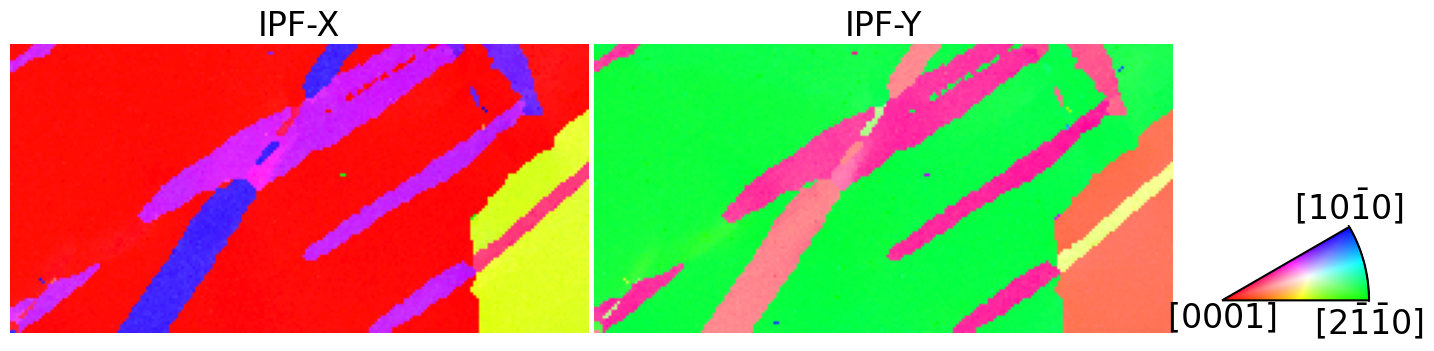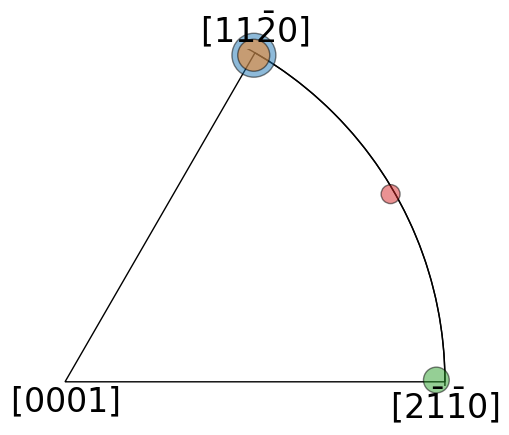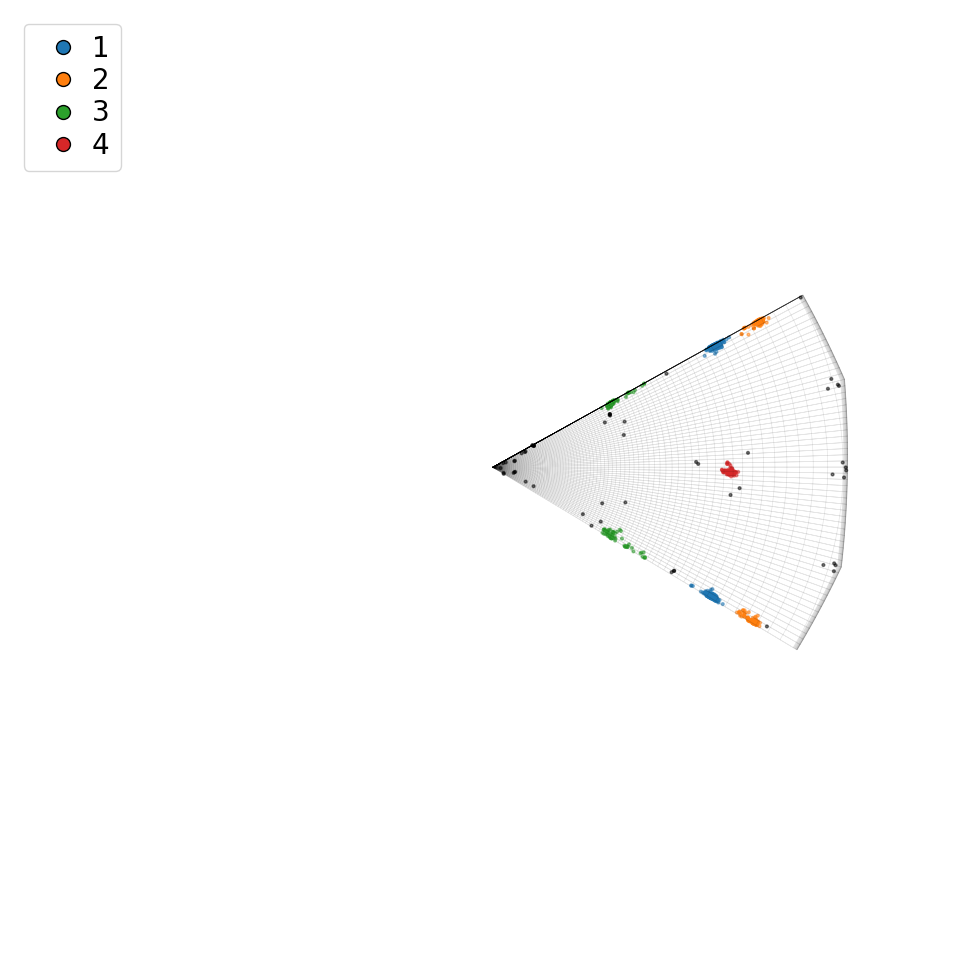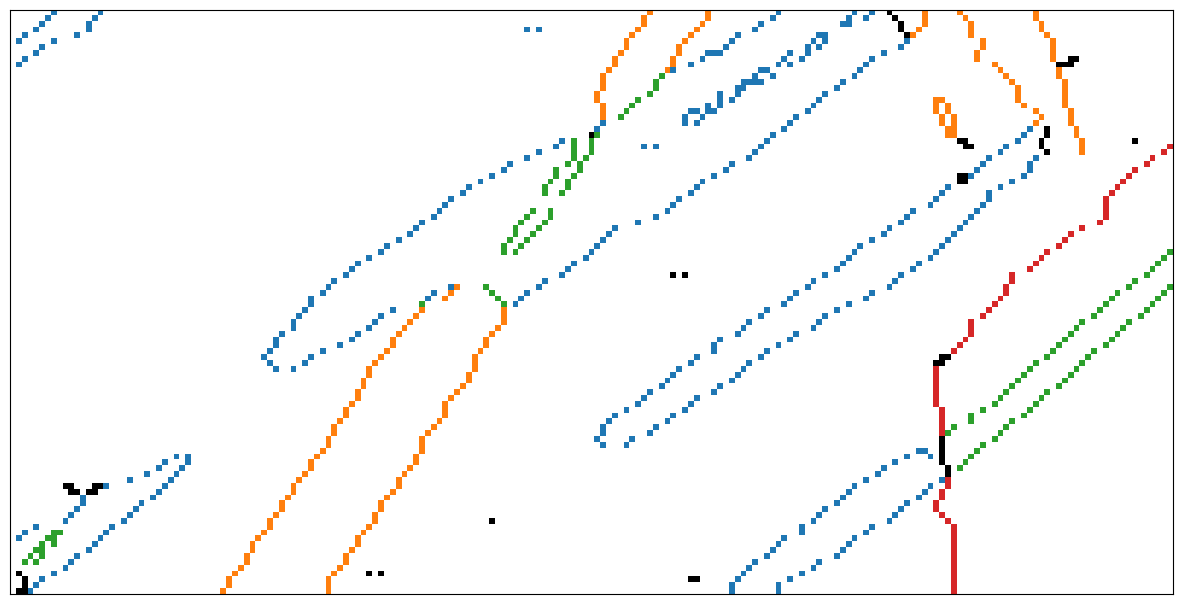# Clustering misorientations#

In this tutorial we will cluster Ti crystal misorientations using data obtained from a highly deformed specimen, using EBSD, as presented in . The data can be downloaded to your local cache via the orix.data module.

Import orix classes and various dependencies

:

# Exchange "inline" for "notebook" (or "qt5" from pyqt) for interactive plotting
%matplotlib inline

from matplotlib.colors import to_rgb
from matplotlib.lines import Line2D
import matplotlib.pyplot as plt
import numpy as np
from skimage.color import label2rgb
from sklearn.cluster import DBSCAN

from orix import data, plot
from orix.quaternion import Orientation, Misorientation, Rotation
from orix.quaternion.symmetry import D6
from orix.vector import Vector3d

plt.rcParams.update({"font.size": 20, "figure.figsize": (10, 10)})


## Import data#

:

ori = data.ti_orientations(allow_download=True)
ori

:

Orientation (193167,) 622
[[ 0.3027  0.0869 -0.5083  0.8015]
[ 0.3088  0.0868 -0.5016  0.8034]
[ 0.3057  0.0818 -0.4995  0.8065]
...
[ 0.4925 -0.1633 -0.668   0.5334]
[ 0.4946 -0.1592 -0.6696  0.5307]
[ 0.4946 -0.1592 -0.6696  0.5307]]


The orientations define transformations from the sample (lab) to the crystal reference frame, i.e. the Bunge convention. The above referenced paper assumes the opposite convention, which is the one used in MTEX. So, we have to invert the orientations

:

ori = ~ori


Reshape the orientation mapping data to the correct spatial dimension for the scan

:

ori = ori.reshape(381, 507)


Select a subset of the orientations with a suitable size for this demonstration

:

ori = ori[-100:, :200]


Plot orientation maps

:

ckey = plot.IPFColorKeyTSL(D6)

directions = [(1, 0, 0), (0, 1, 0)]
titles = ["X", "Y"]

fig, axes = plt.subplots(ncols=2, figsize=(15, 10))
for i, ax in enumerate(axes):
ckey.direction = Vector3d(directions[i])
# Invert because orix assumes lab2crystal when coloring orientations
ax.imshow(ckey.orientation2color(~ori))
ax.set_title(f"IPF-{titles[i]}")
ax.axis("off")

[0.932, 0.37, 0.1, 0.1],  # (Left, bottom, width, height)
projection="ipf",
symmetry=ori.symmetry.laue,
)
ax_ipfkey.plot_ipf_color_key()
ax_ipfkey.set_title("")Map the orientations into the fundamental zone (find symmetrically equivalent orientations with the smallest angle of rotation) of D6

:

ori = ori.map_into_symmetry_reduced_zone()


Compute misorientations (in the horizontal direction)

:

mori_all = Misorientation(~ori[:, :-1] * ori[:, 1:])


Keep only misorientations with a disorientation angle higher than 7$$^{\circ}$$, assumed to represent grain boundaries

:

boundary_mask = mori_all.angle > np.deg2rad(7)


Map the misorientations into the fundamental zone of (D6, D6)

:

mori.symmetry = (D6, D6)
mori = mori.map_into_symmetry_reduced_zone()


## Compute distance matrix#

:

# Increase the chunk size for a faster but more memory intensive computation
D = mori.get_distance_matrix()

[########################################] | 100% Completed | 35.64 s


## Clustering#

:

small_mask = mori.angle < np.deg2rad(7)


For parameter explanations of the DBSCAN algorithm (Density-Based Spatial Clustering for Applications with Noise), see the scikit-learn documentation.

:

# Compute clusters
dbscan = DBSCAN(eps=0.05, min_samples=10, metric="precomputed").fit(D)

unique_labels, all_cluster_sizes = np.unique(
dbscan.labels_, return_counts=True
)
print("Labels:", unique_labels)

n_clusters = unique_labels.size - 1
print("Number of clusters:", n_clusters)

Labels: [-1  0  1  2  3]
Number of clusters: 4


Calculate the mean misorientation associated with each cluster

:

unique_cluster_labels = unique_labels[
1:
]  # Without the "no-cluster" label -1
cluster_sizes = all_cluster_sizes[1:]

rc = Rotation.from_axes_angles((0, 0, 1), 15, degrees=True)

mori_mean = []
for label in unique_cluster_labels:
# Rotate
mori_i = rc * mori[dbscan.labels_ == label]

# Map into the fundamental zone
mori_i.symmetry = (D6, D6)
mori_i = mori_i.map_into_symmetry_reduced_zone()

# Get the cluster mean
mori_i = mori_i.mean()

# Rotate back and add to list
cluster_mean_local = (~rc) * mori_i
mori_mean.append(cluster_mean_local)

cluster_means = Misorientation.stack(mori_mean).flatten()

# Map into the fundamental zone
cluster_means.symmetry = (D6, D6)
cluster_means = cluster_means.map_into_symmetry_reduced_zone()
cluster_means

:

Misorientation (4,) 622, 622
[[ 0.8467  0.2664  0.4606  0.0037]
[-0.7858 -0.3094 -0.5355 -0.0044]
[-0.9515 -0.3075 -0.0015 -0.007 ]
[ 0.8656  0.4338  0.2501  0.0055]]


Inspect misorientations in the axis-angle representation

:

cluster_means.axis

:

Vector3d (4,)
[[0.5006 0.8656 0.007 ]
[0.5003 0.8658 0.0072]
[0.9997 0.0048 0.0228]
[0.8663 0.4994 0.0109]]

:

np.rad2deg(cluster_means.angle)

:

array([64.29349881, 76.40971082, 35.82903611, 60.10115054])


Define reference misorientations associated with twinning orientation relationships

:

# From Krakow et al.
twin_theory = Rotation.from_axes_angles(
axes=[
(1, 0, 0),  # sigma7a
(1, 0, 0),  # sigma11a
(2, 1, 0),  # sigma11b
(1, 0, 0),  # sigma13a
(2, 1, 0),  # sigma13b
],
angles=[64.40, 34.96, 85.03, 76.89, 57.22],
degrees=True,
)


Calculate difference, defined as minimum rotation angle, between measured and theoretical values

:

mori2 = (~twin_theory).outer(cluster_means)
sym_op = D6.outer(D6).unique()
mori2_equiv = (
D6.outer(~twin_theory).outer(sym_op).outer(cluster_means).outer(D6)
)
D2 = mori2_equiv.angle.min(axis=(0, 2, 4))

:

np.rad2deg(D2)

:

array([[ 0.44196598, 12.01907556, 28.59166542, 31.00602591],
[29.33528603, 41.45127188,  1.18969475, 34.15185331],
[37.89471722, 35.23189781, 54.83306878, 25.25351347],
[12.60503699,  0.70196626, 41.07796156, 37.24075935],
[27.53333357, 34.58980591, 29.35942936,  4.44471339]])


We see that the first, second, third, and fourth clusters are within $$4.5^{\circ}$$ of $$\Sigma7$$a, $$\Sigma13$$a, $$\Sigma11$$a, and $$\Sigma13$$b, respectively.

## Visualisation#

Associate colours with clusters for plotting

:

colors = [to_rgb(f"C{i}") for i in range(cluster_means.size)]
labels_rgb = label2rgb(dbscan.labels_, colors=colors, bg_label=-1)


Inspect misorientation axes of clusters in an inverse pole figure

:

cluster_sizes = all_cluster_sizes[1:]
cluster_sizes_scaled = 1000 * cluster_sizes / cluster_sizes.max()

fig, ax = plt.subplots(
figsize=(5, 5), subplot_kw=dict(projection="ipf", symmetry=D6)
)
ax.scatter(
cluster_means.axis, c=colors, s=cluster_sizes_scaled, alpha=0.5, ec="k"
)Plot a top view of the misorientation clusters within the fundamental zone for the (D6, D6) bicrystal symmetry

:

wireframe_kwargs = dict(
color="black", linewidth=0.5, alpha=0.1, rcount=361, ccount=361
)
fig = mori.scatter(
projection="axangle",
wireframe_kwargs=wireframe_kwargs,
c=labels_rgb.reshape(-1, 3),
s=4,
alpha=0.5,
return_figure=True,
)
ax = fig.axes
ax.view_init(elev=90, azim=-60)

handle_kwds = dict(marker="o", color="none", markersize=10)
handles = []
for i in range(n_clusters):
line = Line2D(
, , label=i + 1, markerfacecolor=colors[i], **handle_kwds
)
handles.append(line)
ax.legend(
handles=handles,
loc="upper left",
numpoints=1,
labelspacing=0.15,
columnspacing=0.15,
);Plot side view of misorientation clusters in the fundamental zone for the (D6, D6) bicrystal symmetry

:

fig2 = mori.scatter(
return_figure=True,
wireframe_kwargs=wireframe_kwargs,
c=labels_rgb.reshape(-1, 3),
s=4,
)
ax2 = fig2.axes
ax2.view_init(elev=0, azim=-60)Generate map of boundaries colored according to cluster membership

:

mapping = np.ones(mori_all.shape + (3,))
)

:

fig3, ax3 = plt.subplots(figsize=(15, 10))